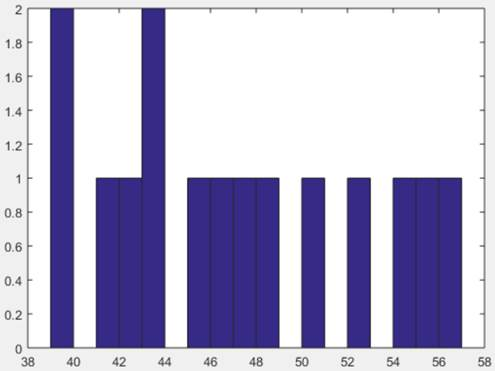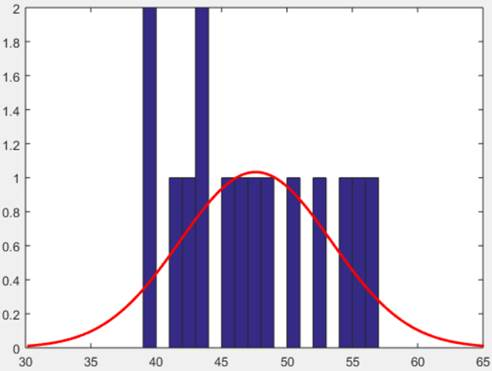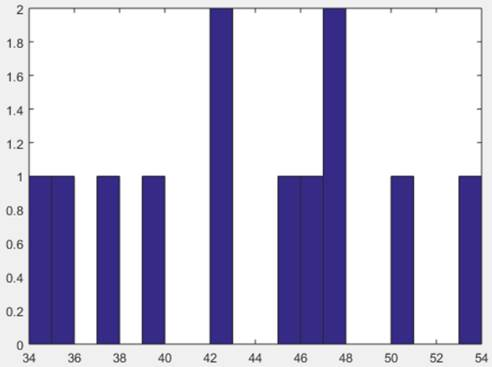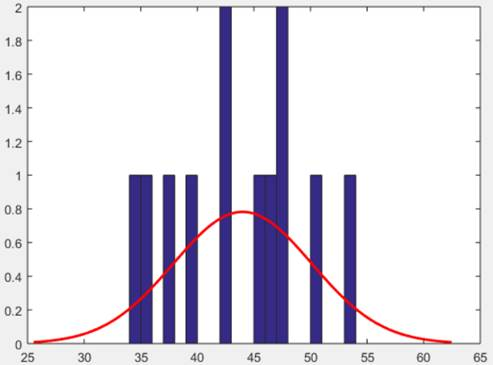# 一、题概述和分析

## （1）实验内容说明：

（验证性实验）某地某年高考后随机抽得15名男生、12名女生的物理考试成绩如下:

男生: 49 48 47 53 51 43 39 57 56 46 42 44 55 44 40



# 二、实验设计总体思路

## 2.2、实验主题部分

### 2.2.1、实验设计思路

#### 2、实现方法

##### （2）检验两样本总体方差是否相等

$H_0':\sigma^2_1=\sigma^2_2$

F没有落在拒绝域中，故接受$H_0$，认为两样本总体方差相等。

##### （3）检验两样本总体均值是否相等

$H_0:\mu1=\mu2$,$H_1:\mu1\neq\mu2$

$$S^2_w=\frac{(n_1-1)S^2_1+(n_2-1)S^2_2}{n_1+n_2-2}$$

t没有落在拒绝域中，故接受$H_0$，认为这个地区男女生的物理考试成绩不相上下

### 2.2.2、实验结果及分析

X的频数直方图和正态曲线拟合：Y的频数直方图和正态曲线拟合：### 2.2.3、程序及其说明

 1 2 3 4 5 6 7 8  X=[49,48,47,53,51,43,39,57,56,46,42,44,55,44,40]; %将男生成绩的样本定义为X hist(X,18); %绘制频数直方图 histfit(X,18); %观察正态曲线拟合情况 [muhat,sigmahat,muci,sigmaci]=normfit(X,0.05); %将女生成绩的样本定义为X Y=[46,40,47,51,43,36,43,38,48,54,48,34]; %参数估计 hist(Y,20); %绘制频数直方图 histfit(Y,20); %观察正态曲线拟合情况 [muhat,sigmahat,muci,sigmaci]=normfit(Y,0.05); %参数估计 
 1  F=5.7916/6.12=0.9463 %计算 
 1  T=3.6/(5.936*(1/15+1/12)^0.5)=1.5659 %计算